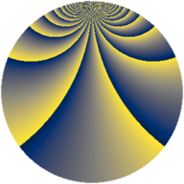# Properties

 Label 2760.2.dgLevel $2760$ Weight $2$ Character orbit 2760.dg Rep. character $\chi_{2760}(37,\cdot)$ Character field $\Q(\zeta_{44})$ Dimension $5760$ Sturm bound $1152$

# Related objects

## Defining parameters

 Level: $$N$$ $$=$$ $$2760 = 2^{3} \cdot 3 \cdot 5 \cdot 23$$ Weight: $$k$$ $$=$$ $$2$$ Character orbit: $$[\chi]$$ $$=$$ 2760.dg (of order $$44$$ and degree $$20$$) Character conductor: $$\operatorname{cond}(\chi)$$ $$=$$ $$920$$ Character field: $$\Q(\zeta_{44})$$ Sturm bound: $$1152$$

## Dimensions

The following table gives the dimensions of various subspaces of $$M_{2}(2760, [\chi])$$.

Total New Old
Modular forms 11680 5760 5920
Cusp forms 11360 5760 5600
Eisenstein series 320 0 320

## Trace form

 $$5760q - 8q^{6} + O(q^{10})$$ $$5760q - 8q^{6} + 8q^{16} + 32q^{31} + 40q^{32} - 8q^{36} - 220q^{42} - 48q^{46} + 32q^{48} - 32q^{50} + 108q^{52} + 32q^{58} - 112q^{62} - 32q^{70} + 396q^{80} + 576q^{81} + 40q^{82} + 24q^{92} + 88q^{96} - 56q^{98} + O(q^{100})$$

## Decomposition of $$S_{2}^{\mathrm{new}}(2760, [\chi])$$ into newform subspaces

The newforms in this space have not yet been added to the LMFDB.

## Decomposition of $$S_{2}^{\mathrm{old}}(2760, [\chi])$$ into lower level spaces

$$S_{2}^{\mathrm{old}}(2760, [\chi]) \cong$$ $$S_{2}^{\mathrm{new}}(920, [\chi])$$$$^{\oplus 2}$$